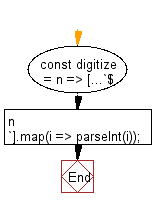# JavaScript: Converts a specified number to an array of digits

## JavaScript fundamental (ES6 Syntax): Exercise-7 with Solution

Write a JavaScript program to convert a specified number into an array of digits.

Note: Convert the number to a string, using the spread operator (...) to build an array.

• Use Math.abs() to strip the number's sign.
• Convert the number to a string, using the spread operator (...) to build an array.
• Use Array.prototype.map() and parseInt() to transform each value to an integer.

Sample Solution:

JavaScript Code:

``````const digitize = n => [...`\${n}`].map(i => parseInt(i));

console.log(digitize(123));
console.log(digitize(1230));
```
```

Sample Output:

```[1,2,3]
[1,2,3,0]
```

Pictorial Presentation:Flowchart:Live Demo:

See the Pen javascript-basic-exercise-1-7 by w3resource (@w3resource) on CodePen.

Improve this sample solution and post your code through Disqus

What is the difficulty level of this exercise?

Test your Programming skills with w3resource's quiz.

﻿